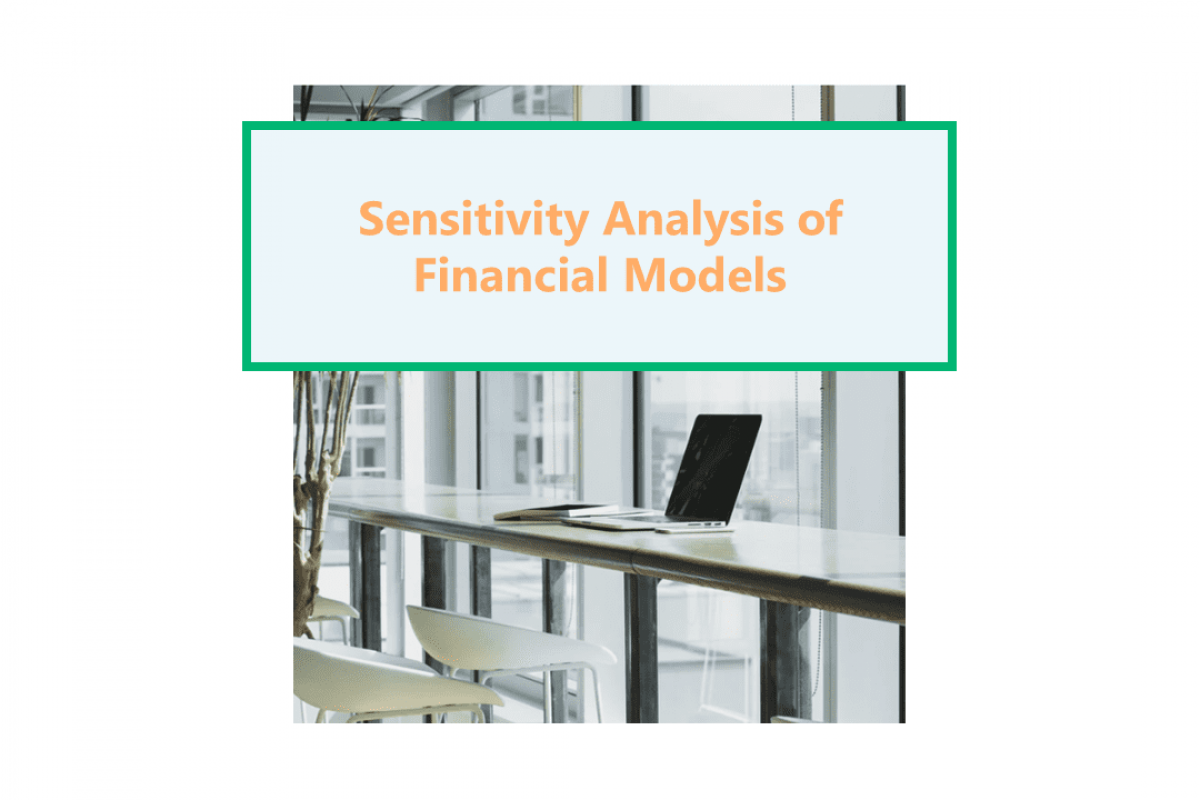# Sensitivity Analysis of Financial Models (What-If Analysis)Keyskillset
13
February
2020### What is Sensitivity Analysis?

Sensitivity analysis determines how different values of an independent variable affect a dependent variable under a given set of assumptions. People use it in the business world and in the field of finance. Besides, financial analysts and economists use it widely in their analysis. Meanwhile, some people know it as a what-if analysis.

We use Sensitivity analysis in financial modeling to analyze how different values of a set of independent variables affect a specific dependent variable. All of this occurs under certain specific conditions. In general, companies use sensitivity analysis in a wide range of fields, ranging from biology and geography to economics and engineering.

### Sensitivity Analysis in Excel

In our example m#, we will evaluate how different assumptions for Revenue Growth and Cost of Revenue (% of sales) in 2019 will affect Equity Value per Share.

In our Data Table, 14.3% is the Revenue Growth and 35.4% is the Cost of Revenue (% of sales). It’s an input, not links. Let’s see how these values would affect Equity Value per Share.

In G36 we should put a link to the cell in our model that we want to track. The value changes when revenue growth and/or cost of the revenue goes up and/or down from our scenario. In our example, it’s Equity Value per Share (cell B42).

From here, highlight the whole data table including the outside (G36 – L41). For that, use Excel shortcuts Ctrl + Shift + Right Arrow and then Ctrl + Shift + Down Arrow.

To access the What-If Analysis -> Data Table on the Ribbon, use Excel shortcut Alt A W T.

In the data table the row input cell is Cost of Revenue (% of sales), cell F28. Column input cell is the Revenue Growth for our example, cell F27.

Our data table is ready. Sometimes you need to click F9 (Function 9 on the top of your keyboard) to recalculate the table. But also, to get the correct results (in case if your settings say “Calculate Automatically except for data tables”).

In our data table, we got \$138.6 as the Equity Value per Share. Our model’s assumptions were for the Revenue Growth of 14.3% and the Cost of Revenue (% of sales) of 35.4%.

In case the Revenue Growth was 15.3% and Cost of Revenue (% of sales) was 36.4% – the Equity Value per Share is equal to \$141.2.

### Conditional Formatting

Conditional formatting makes it easy to highlight interesting cells or ranges of cells. It emphasizes unusual values. Moreover, it helps to visualize data by using data bars, color scales, and icon sets. These correspond to specific variations in the data.

A conditional format changes the appearance of cells on the basis of conditions that you specify. If the conditions are true, we will format the cell range. Otherwise, if the conditions are false, we don’t format the cell range. There are many built-in conditions, and you can also create your own (including by using a formula that evaluates to True or False).

Let’s use Conditional Formatting to help you visually explore and analyze data. For this example, highlight the insight of the data table (H37 – L 31). Then, access Conditional Formatting on the Ribbon with Excel shortcut Alt H L, N for the new rule.

Let’s apply this color to fill on the screenshot to the highlighted cells with the value between \$130 and \$150.

### Sensitivity Analysis vs. Scenario Analysis

Sensitivity analysis is the study of how the outcome of a decision changes due to variations in input. We use it in situations that rely on one or more input variables.  In contrast,  Scenario analysis is the process of predicting the future value of an investment. It depends on changes that may occur to existing variables. It requires one to explore the impact of different market conditions on the project or the whole investment. Learn more about Scenario Analysis in our article.

### Conclusion

Sensitivity analysis determines how different values of an independent variable affect a particular dependent variable under a given set of assumptions.

1. We also call this model a what-if or simulation analysis.
2. It acts as an in-depth study of all the variables.
3. Decision-makers can find where they need to improve.